Image recognition basics

There is multiple image classification datasets available online or embedded in python ML related modules, and this notebook contains just a sample code for image classification on those publicly available datasets. In this post, I will just use a very ‘blond’ solution and definitely not a perfect one (deep neural networks – those are much better algorithms for those kind of problems, but they will be covered in a separate post). Idea was to define a common pattern of code for such problems (if something like that exists at all..), learn how to modify/display image datasets and how to apply ML classification algorithms on them. First two examples are based on Python Data Science Handbook by Jake VanderPlas, which in my humble opinion is one of the best sources for studying ML python modules, and what it is really awesome , this book is available it Jupyter notebook format, so you can take a code and experiment with it. Although my code might look different, than the one in this position, it is was based on Jakes book, so all credits go to him, thus I’m including here following comment:

“….. Python Data Science Handbook by Jake VanderPlas; the content is available on GitHub.*
The text is released under the CC-BY-NC-ND license, and code is released under the MIT license. If you find this content useful, please consider supporting the work by buying the book!….”

Third part of my code is a simple solution of a problem introduced in Udacity nanodegree program, but as unfortunately I didn’t participate in this program, training\test datasets were downloaded from German Traffic Sign Dataset. If you want to dive deeper into those 3 problems, I would recommend you to get more info directly from those mentioned above sources.

from sklearn.datasets import load_digits
from sklearn.model_selection import train_test_split
from sklearn.ensemble import RandomForestClassifier
from sklearn import metrics
import matplotlib.pyplot as plt
import numpy as np
import pandas as pd
from random import randint
%matplotlib inline
import seaborn as sns
import warnings
warnings.filterwarnings("ignore");

Digits recognition

#Lets take a look at this dataset
Help on function load_digits in module sklearn.datasets.base:

Load and return the digits dataset (classification).

Each datapoint is a 8x8 image of a digit.

=================   ==============
Classes                         10
Samples per class             ~180
Samples total                 1797
Dimensionality                  64
Features             integers 0-16
=================   ==============

Read more in the :ref:User Guide <datasets>.

Parameters
----------
n_class : integer, between 0 and 10, optional (default=10)
The number of classes to return.

return_X_y : boolean, default=False.
If True, returns (data, target) instead of a Bunch object.
See below for more information about the data and target object.

Returns
-------
data : Bunch
Dictionary-like object, the interesting attributes are:
'data', the data to learn, 'images', the images corresponding
to each sample, 'target', the classification labels for each
sample, 'target_names', the meaning of the labels, and 'DESCR',
the full description of the dataset.

(data, target) : tuple if return_X_y is True

Examples
--------
To load the data and visualize the images::

>>> from sklearn.datasets import load_digits
>>> digits = load_digits()
>>> print(digits.data.shape)
(1797, 64)
>>> import matplotlib.pyplot as plt #doctest: +SKIP
>>> plt.gray() #doctest: +SKIP
>>> plt.matshow(digits.images) #doctest: +SKIP
>>> plt.show() #doctest: +SKIP

#Check datset size
print(digits.data.shape)
(1797, 64)
#Each datapoint is a 8x8 image, with pixel value between 0 and 16
digits.images
array([[  0.,   0.,   7.,  15.,  13.,   1.,   0.,   0.],
[  0.,   8.,  13.,   6.,  15.,   4.,   0.,   0.],
[  0.,   2.,   1.,  13.,  13.,   0.,   0.,   0.],
[  0.,   0.,   2.,  15.,  11.,   1.,   0.,   0.],
[  0.,   0.,   0.,   1.,  12.,  12.,   1.,   0.],
[  0.,   0.,   0.,   0.,   1.,  10.,   8.,   0.],
[  0.,   0.,   8.,   4.,   5.,  14.,   9.,   0.],
[  0.,   0.,   7.,  13.,  13.,   9.,   0.,   0.]])
#Training dataset are flattened image arrays (64X1)
digits.data
array([  0.,   0.,   7.,  15.,  13.,   1.,   0.,   0.,   0.,   8.,  13.,
6.,  15.,   4.,   0.,   0.,   0.,   2.,   1.,  13.,  13.,   0.,
0.,   0.,   0.,   0.,   2.,  15.,  11.,   1.,   0.,   0.,   0.,
0.,   0.,   1.,  12.,  12.,   1.,   0.,   0.,   0.,   0.,   0.,
1.,  10.,   8.,   0.,   0.,   0.,   8.,   4.,   5.,  14.,   9.,
0.,   0.,   0.,   7.,  13.,  13.,   9.,   0.,   0.])
#Let's plot sample digit
plt.figure(figsize=(6,3))
plt.matshow(digits.images, fignum=1);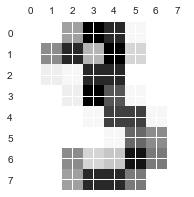# Displaying first 64 images with target values

fig, axes =plt.subplots(8, 8, figsize=(8, 8),
subplot_kw={'xticks':[], 'yticks':[]},
gridspec_kw=dict(hspace=0.1, wspace=0.1));

for i, ax in enumerate(axes.flat):
ax.imshow(digits.images[i], cmap='binary')
ax.text(0, 7, str(digits.target[i]),color='green')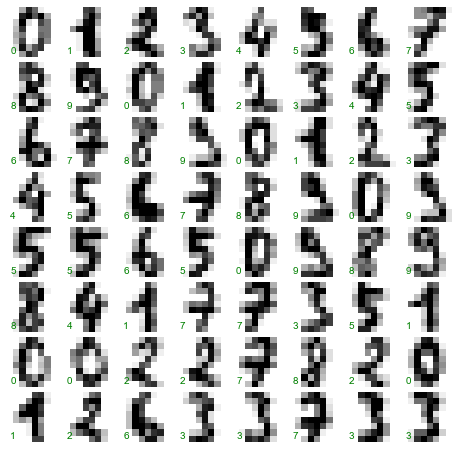#Splitiing datset on training (80%) and testing (20%) dataset
Xtrain, Xtest, ytrain, ytest = train_test_split(digits.data, digits.target, test_size=0.8, random_state=1000)

Random forest are basically a collection of a number of decision trees and together they are used to give the final output. Like, decision trees random forest is also a supervised learning algorithm which can be used for both regression and classification problems. To get the prediction from a random forest, we use the output from each of the trees which we commonly call as “votes”. The final output is the one which has the most number of votes. (source: https://analyticsdefined.com/introduction-random-forests/)

# Random Forest classifier
model=RandomForestClassifier(n_estimators=1000)
model.fit(Xtrain,ytrain)
ypred=model.predict(Xtest)

Classifier output quality evaluation

# Displaying results. If digit in bottom left corner is green then classification was correct.
#If this digit is red, then classification was wrong.

fig, axes =plt.subplots(8,8, figsize=(8, 8),
subplot_kw={'xticks':[], 'yticks':[]},
gridspec_kw=dict(hspace=0.1, wspace=0.1));

for i, ax in enumerate(axes.flat):
ax.imshow(Xtest[i].reshape((8,8)), cmap='binary')
if ytest[i]== ypred[i]:
ax.text(0, 7, str(ypred[i]),color='green')
else:
ax.text(0, 7, str(ypred[i]),color='red')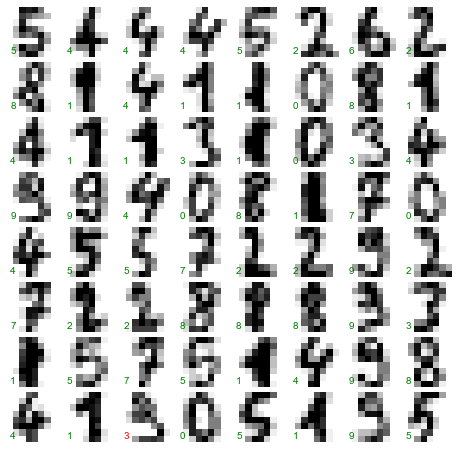# Display calssification report
print(metrics.classification_report(ypred, ytest))
precision    recall  f1-score   support

0       0.99      0.96      0.98       142
1       0.99      0.92      0.95       158
2       0.99      0.99      0.99       139
3       0.89      0.96      0.92       140
4       0.92      0.94      0.93       144
5       0.95      0.94      0.95       141
6       0.97      0.97      0.97       147
7       0.96      0.90      0.93       148
8       0.90      0.89      0.90       140
9       0.88      0.96      0.92       139

avg / total       0.94      0.94      0.94      1438

Quick theory reminder:

A true positive (TP) is an outcome where the model correctly predicts the positive class. Similarly, a true negative (TN) is an outcome where the model correctly predicts the negative class.

A false positive (FP)is an outcome where the model incorrectly predicts the positive class. And a false negative (FN) is an outcome where the model incorrectly predicts the negative class.

Precision shows what proportion of positive identifications was actually correct:

$$Precision= \frac{TP}{TP+FP}$$

Recall shows what proportion of actual positives was identified correctly

$$Recall= \frac{TP}{TP+FN}$$

Accuracy is one metric for evaluating classification models. Informally, accuracy is the fraction of predictions our model got right. Formally, accuracy has the following definition:

$$Accuracy= \frac{Number of correct predictions}{Total number of predictions}$$
so:
$$Accuracy= \frac{TP+TN}{TP+TN+FP+FN}$$

Another metric for evaluating classification models is f1-score

$$f1-score= \frac{2*Precision*Recall}{Precision+Recall}$$

Faces recognition

from sklearn.datasets import fetch_lfw_people
#Lets take a look at this dataset
help(fetch_lfw_people)
Help on function fetch_lfw_people in module sklearn.datasets.lfw:

fetch_lfw_people(data_home=None, funneled=True, resize=0.5, min_faces_per_person=0, color=False, slice_=(slice(70, 195, None), slice(78, 172, None)), download_if_missing=True)
Loader for the Labeled Faces in the Wild (LFW) people dataset

This dataset is a collection of JPEG pictures of famous people
collected on the internet, all details are available on the
official website:

http://vis-www.cs.umass.edu/lfw/

Each picture is centered on a single face. Each pixel of each channel
(color in RGB) is encoded by a float in range 0.0 - 1.0.

The task is called Face Recognition (or Identification): given the
picture of a face, find the name of the person given a training set
(gallery).

The original images are 250 x 250 pixels, but the default slice and resize
arguments reduce them to 62 x 74.

Parameters
----------
data_home : optional, default: None
Specify another download and cache folder for the datasets. By default
all scikit learn data is stored in '~/scikit_learn_data' subfolders.

funneled : boolean, optional, default: True
Download and use the funneled variant of the dataset.

resize : float, optional, default 0.5
Ratio used to resize the each face picture.

min_faces_per_person : int, optional, default None
The extracted dataset will only retain pictures of people that have at
least min_faces_per_person different pictures.

color : boolean, optional, default False
Keep the 3 RGB channels instead of averaging them to a single
gray level channel. If color is True the shape of the data has
one more dimension than the shape with color = False.

slice_ : optional
Provide a custom 2D slice (height, width) to extract the
'interesting' part of the jpeg files and avoid use statistical
correlation from the background

If False, raise a IOError if the data is not locally available
instead of trying to download the data from the source site.

Returns
-------
dataset : dict-like object with the following attributes:

dataset.data : numpy array of shape (13233, 2914)
Each row corresponds to a ravelled face image of original size 62 x 47
pixels. Changing the slice_ or resize parameters will change the
shape of the output.

dataset.images : numpy array of shape (13233, 62, 47)
Each row is a face image corresponding to one of the 5749 people in
the dataset. Changing the slice_ or resize parameters will change
the shape of the output.

dataset.target : numpy array of shape (13233,)
Labels associated to each face image. Those labels range from 0-5748
and correspond to the person IDs.

dataset.DESCR : string
Description of the Labeled Faces in the Wild (LFW) dataset.

#Extract only those faces for which we have at least 100 pictures
faces = fetch_lfw_people(min_faces_per_person=100)
print(faces.target_names)
print(faces.images.shape)
['Colin Powell' 'Donald Rumsfeld' 'George W Bush' 'Gerhard Schroeder'
'Tony Blair']
(1140, 62, 47)
#Each datapoint is a 62x47 image, with pixel value between 0 and 255.
faces.images
array([[  52.33333206,   49.33333206,   69.33333588, ...,   83.        ,
48.33333206,   37.66666794],
[  42.        ,   46.        ,   71.        , ...,  119.        ,
76.33333588,   51.        ],
[  38.        ,   50.33333206,   78.66666412, ...,  145.        ,
107.        ,   68.        ],
...,
[ 138.        ,  112.33333588,   67.66666412, ...,  229.        ,
225.33332825,  218.        ],
[ 127.33333588,   91.33333588,   58.66666794, ...,  233.        ,
227.        ,  221.        ],
[ 108.66666412,   73.        ,   57.        , ...,  235.66667175,
228.66667175,  222.        ]], dtype=float32)
#Let's plot sample digit
plt.figure(figsize=(6,3))
plt.matshow(faces.images, fignum=1);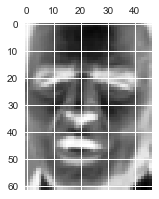# Displaying first 16 images with target names

fig, axes =plt.subplots(4, 4, figsize=(8, 12),
subplot_kw={'xticks':[], 'yticks':[]},
gridspec_kw=dict(hspace=0.1, wspace=0.1));

for i, ax in enumerate(axes.flat):
ax.imshow(faces.images[i],'bone')
ax.text(0, 67, faces.target_names[faces.target[i]],color='green')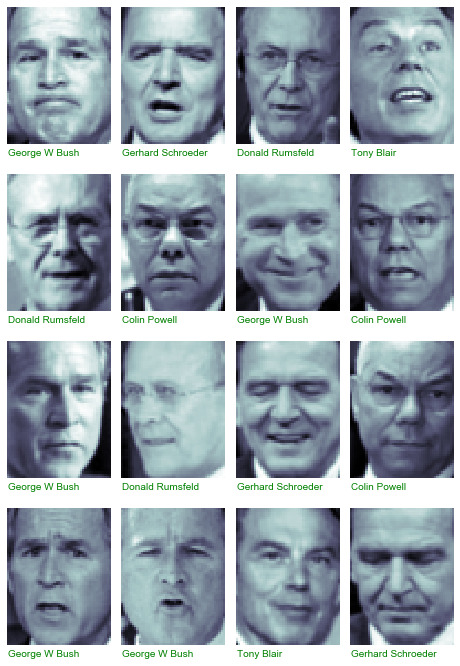from sklearn.svm import SVC
from sklearn.decomposition import PCA
from sklearn.pipeline import make_pipeline

In order to detremine number of component lets plot “cumulative explained variance ratio as a function of the number of components”.

pca = PCA().fit(faces.data)
plt.figure(figsize=(10,10))
plt.plot(np.cumsum(pca.explained_variance_ratio_))
plt.xlabel('number of components')
plt.ylabel('cumulative explained variance');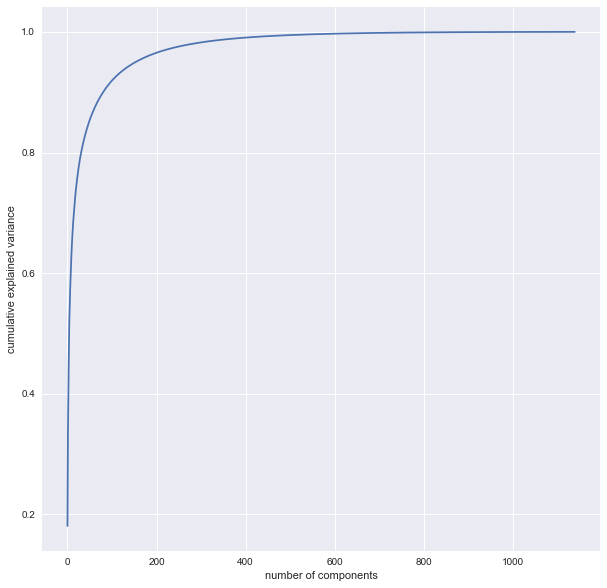Lets use 200 components, as it contains approximately 95% of the variance

pca = PCA(n_components=200, whiten=True)
svc = SVC(kernel='rbf')
model_pipe = make_pipeline(pca, svc)
from sklearn.model_selection import train_test_split, GridSearchCV
Xtrain, Xtest, ytrain, ytest = train_test_split(faces.data, faces.target, test_size=0.2)
param_grid = {'svc__C': [1, 5, 10, 50],
'svc__gamma': [0.0001, 0.0005, 0.001, 0.005]}
grid = GridSearchCV(model_pipe, param_grid)
grid.fit(Xtrain, ytrain)
print(grid.best_params_)
{'svc__C': 10, 'svc__gamma': 0.0005}
ypred = grid.best_estimator_.predict(Xtest)
# Displaying results. If name in bottom left corner is green then classification was correct.
#If this name is red, then classification was wrong.

fig, axes =plt.subplots(4,4, figsize=(8, 12),
subplot_kw={'xticks':[], 'yticks':[]},
gridspec_kw=dict(hspace=0.1, wspace=0.1));

for i, ax in enumerate(axes.flat):
ax.imshow(Xtest[i].reshape((62,47)), cmap='bone')
if ytest[i]== ypred[i]:
ax.text(0, 67, faces.target_names[ypred[i]],color='green')
else:
ax.text(0, 67, faces.target_names[ypred[i]],color='red')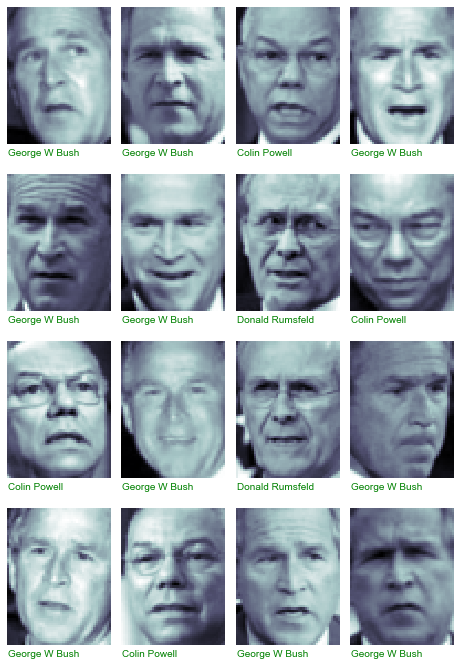from sklearn.metrics import classification_report
print(classification_report(ytest, ypred,
target_names=faces.target_names))
precision    recall  f1-score   support

Colin Powell       0.90      0.90      0.90        48
Donald Rumsfeld       0.94      0.64      0.76        25
George W Bush       0.82      0.95      0.88       107
Gerhard Schroeder       1.00      0.77      0.87        22
Tony Blair       0.86      0.69      0.77        26

avg / total       0.87      0.86      0.86       228

from sklearn.metrics import confusion_matrix
conf_mat = confusion_matrix(ytest, ypred)
sns.heatmap(conf_mat, square=True, annot=True, fmt='d', cbar=True,
xticklabels=faces.target_names,
yticklabels=faces.target_names)
plt.xlabel('Predicted label')
plt.ylabel('True label');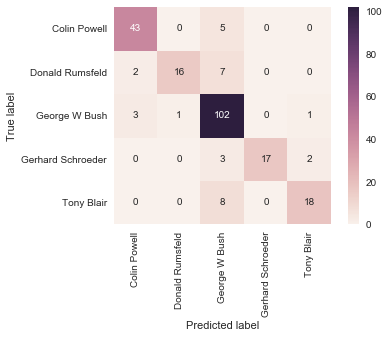Traffic signs recognition

#https://github.com/jeremy-shannon/CarND-Traffic-Sign-Classifier-Project/blob/master/Traffic_Sign_Classifier.md
#import module for working with pickled data
import pickle

Please download data from German Traffic Sign Dataset, as those files are too large to push them to github

training_raw_data=".\\signs\\train.p"
testing_raw_data=".\\signs\\test.p"
with open(training_raw_data, 'rb') as file:
with open(testing_raw_data, 'rb') as file:
print(train['features'].shape)
print(train['labels'].shape)
(39209, 32, 32, 3)
(39209,)
Xtrain=train['features']
ytrain=train['labels']
Xtest=test['features']
ytest=test['labels']
# Function coverting to grayscale and scaling values to 0-1
def rgb2grey(rgb):
#rgb=(0.299 * rgb[:, :, :, 0] + 0.587 * rgb[:, :, :, 1] + 0.114 * rgb[:, :, :, 2])/255.
rgb=(0.299 * rgb[:, :, :, 0] + 0.587 * rgb[:, :, :, 1] + 0.114 * rgb[:, :, :, 2])
return rgb
Xtrain =rgb2grey(Xtrain)
Xtest =rgb2grey(Xtest)
fig, axes =plt.subplots(6,5, figsize=(18, 14),
subplot_kw={'xticks':[], 'yticks':[]},
gridspec_kw=dict(hspace=0.2, wspace=0.1));

for i, ax in enumerate(axes.flat):
rand_num=randint(0, Xtrain.shape)
ax.imshow(Xtrain[rand_num], cmap='bone')
ax.text(0, 34, label_names.iloc[train['labels'][rand_num]]['SignName'],color='green')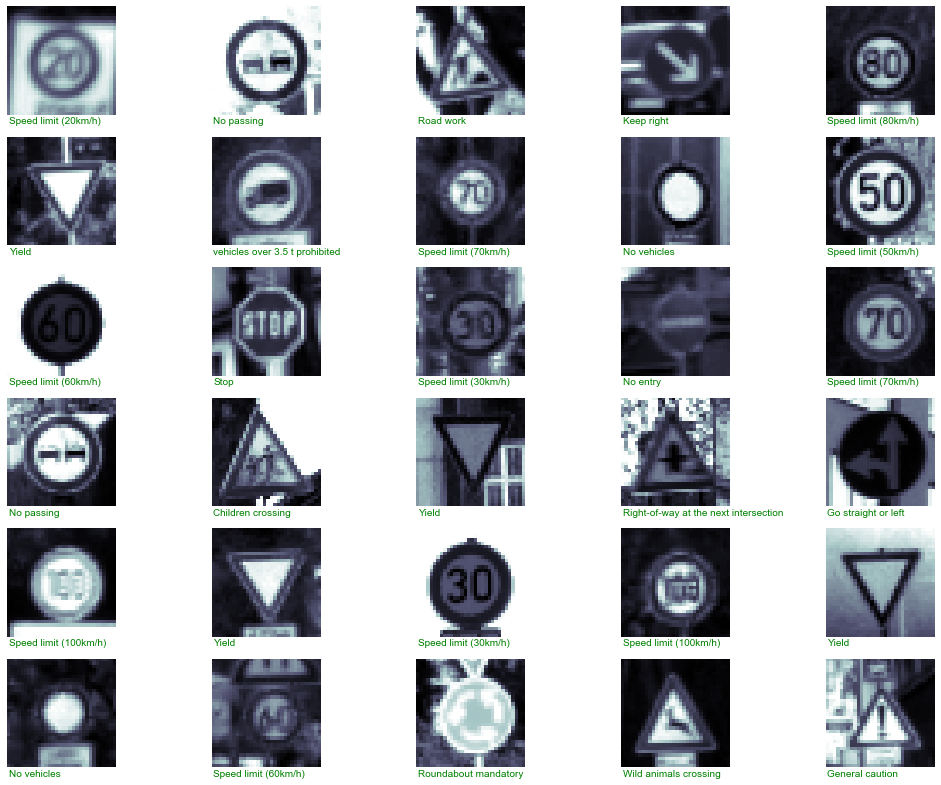Xtrain_data=np.resize(Xtrain, (Xtrain.shape,Xtrain.shape*Xtrain.shape))
Xtest_data=np.resize(Xtest, (Xtest.shape,Xtest.shape*Xtest.shape))
pca = PCA().fit(Xtrain_data)
plt.figure(figsize=(10,10))
plt.plot(np.cumsum(pca.explained_variance_ratio_))
plt.xlabel('number of components')
plt.ylabel('cumulative explained variance');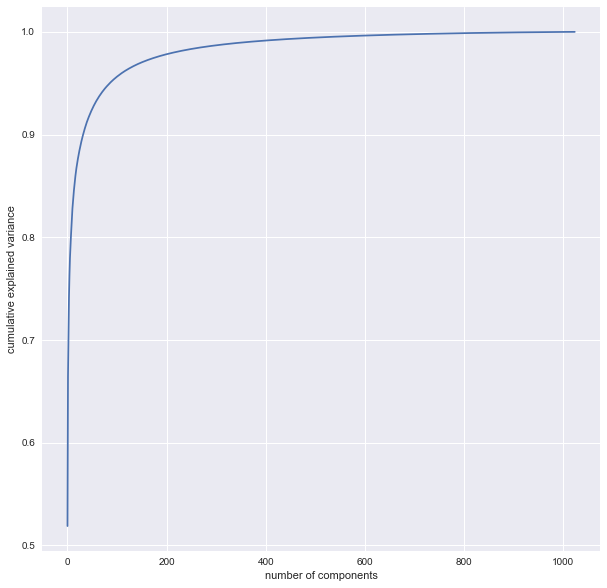Lets use 200 components, as it contains approximately 95% of the variance

pca = PCA(n_components=200, whiten=True)
#{'svc__C': 10, 'svc__gamma': 0.001} from grid search
svc = SVC(kernel='rbf', C=10, gamma=0.001)
model_pipe = make_pipeline(pca, svc)
model_pipe.fit(Xtrain_data, ytrain)
Pipeline(steps=[('pca', PCA(copy=True, iterated_power='auto', n_components=200, random_state=None,
svd_solver='auto', tol=0.0, whiten=True)), ('svc', SVC(C=10, cache_size=200, class_weight=None, coef0=0.0,
decision_function_shape=None, degree=3, gamma=0.001, kernel='rbf',
max_iter=-1, probability=False, random_state=None, shrinking=True,
tol=0.001, verbose=False))])
ypred = model_pipe.predict(Xtest_data)
# Displaying results. If sign name in bottom left corner is green then classification was correct.
#If this name is red, then classification was wrong.

fig, axes =plt.subplots(6,5, figsize=(18, 14),
subplot_kw={'xticks':[], 'yticks':[]},
gridspec_kw=dict(hspace=0.2, wspace=0.1));

for i, ax in enumerate(axes.flat):
rand_num=randint(0, Xtest.shape)
ax.imshow(Xtest[rand_num], cmap='bone')
if ytest[rand_num]== ypred[rand_num]:
ax.text(0, 34, label_names.iloc[ypred[rand_num]]['SignName'],color='green')
else:
ax.text(0, 34, label_names.iloc[ypred[rand_num]]['SignName'],color='red')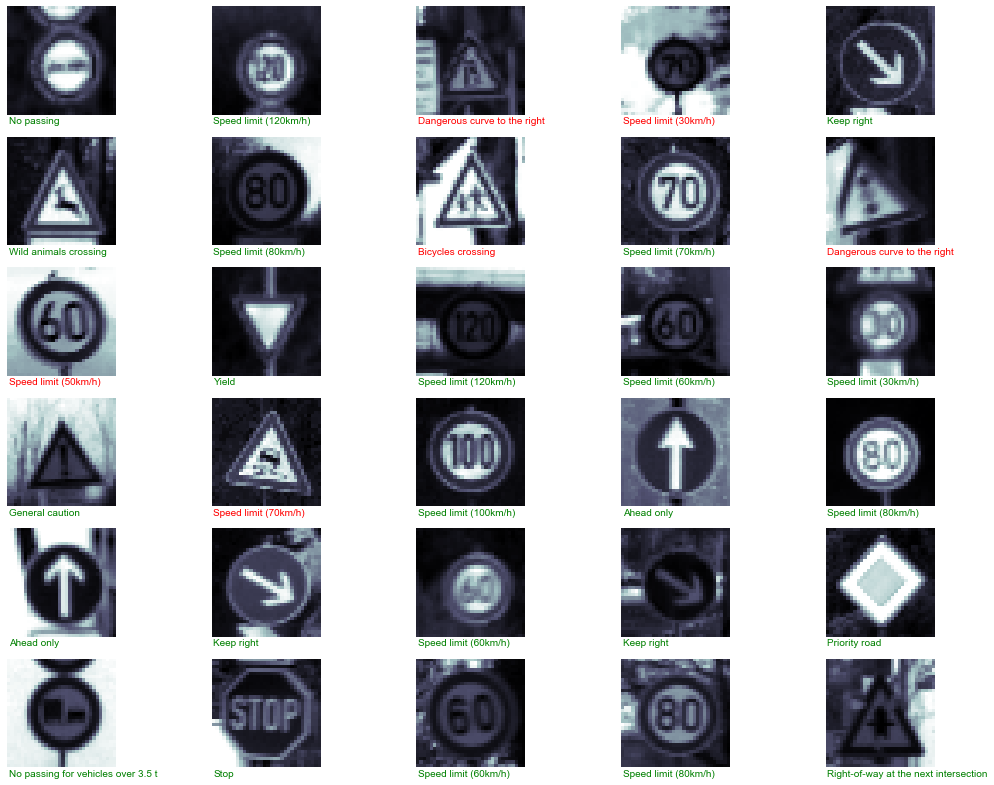from sklearn.metrics import classification_report
print(classification_report(ytest, ypred,
target_names=label_names['SignName']))
precision    recall  f1-score   support

Speed limit (20km/h)       0.69      0.55      0.61        60
Speed limit (30km/h)       0.81      0.87      0.84       720
Speed limit (50km/h)       0.81      0.90      0.85       750
Speed limit (60km/h)       0.67      0.82      0.74       450
Speed limit (70km/h)       0.80      0.85      0.82       660
Speed limit (80km/h)       0.67      0.83      0.74       630
End of speed limit (80km/h)       0.91      0.75      0.82       150
Speed limit (100km/h)       0.88      0.72      0.79       450
Speed limit (120km/h)       0.77      0.82      0.79       450
No passing       0.93      0.80      0.86       480
No passing for vehicles over 3.5 t       0.89      0.95      0.92       660
Right-of-way at the next intersection       0.88      0.86      0.87       420
Priority road       0.78      0.92      0.85       690
Yield       0.96      0.94      0.95       720
Stop       0.88      0.87      0.87       270
No vehicles       0.73      0.74      0.73       210
vehicles over 3.5 t prohibited       0.98      0.93      0.96       150
No entry       0.99      0.87      0.93       360
General caution       0.84      0.60      0.70       390
Dangerous curve to the left       0.59      0.50      0.54        60
Dangerous curve to the right       0.62      0.77      0.69        90
Double curve       0.69      0.70      0.70        90
Bumpy road       0.77      0.97      0.86       120
Slippery road       0.67      0.61      0.64       150
Road narrows on the right       0.55      0.48      0.51        90
Road work       0.91      0.81      0.86       480
Traffic signals       0.86      0.76      0.81       180
Pedestrians       0.77      0.50      0.61        60
Children crossing       0.92      0.54      0.68       150
Bicycles crossing       0.53      0.96      0.68        90
Beware of ice/snow       0.65      0.45      0.54       150
Wild animals crossing       0.82      0.89      0.85       270
End of all speed and passing limits       0.76      0.78      0.77        60
Turn right ahead       0.82      0.96      0.88       210
Turn left ahead       0.91      0.97      0.94       120
Ahead only       0.96      0.77      0.86       390
Go straight or right       0.97      0.90      0.94       120
Go straight or left       0.65      0.70      0.67        60
Keep right       0.98      0.89      0.93       690
Keep left       0.82      0.67      0.74        90
Roundabout mandatory       0.65      0.36      0.46        90
End of no passing       0.65      0.70      0.67        60
End of no passing by vehicles over 3.5 t       0.94      0.73      0.83        90

avg / total       0.84      0.83      0.83     12630

I would say, that model accuracy is so so , but honestly I wouldn’t like to seat in autonomous car with this implementation of traffic sign recognition model. I will try better in another post about deep neural networks.